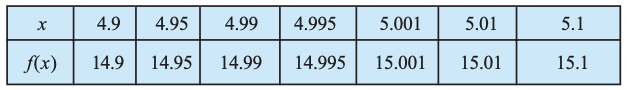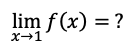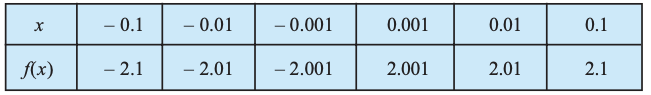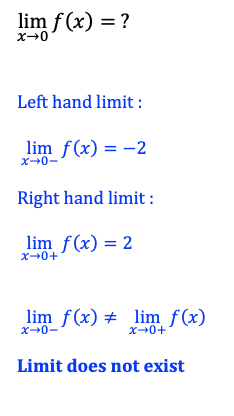# Practice – Find limit of a function from table of values

Questions :

The function f(x) is defined for all real values of x. The values of the function f for a few values of x are given below. Find :The function f(x) is defined for all real values of x. The values of the function f for a few values of x are given below. Find :The function f(x) is defined for all real values of x. The values of the function f for a few values of x are given below. Find :Explanations :